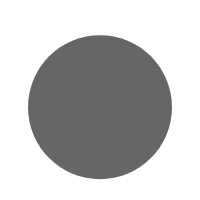# Magic Square

Contents

A magic square is a grid of numbers where each row, column, and diagonal add up to the same number. This number is called the “magic number.” The earliest known magic square is from China, and it dates back to 2200 BCE. It was a 3×3 grid with the numbers 1-9, and the magic number was 15. In this blog post, knowledge glow will explore the types, formulas and magic squares. We’ll also see how they’re used in modern day math and science.

## What is a Magic Square

A magic square is a grid of numbers where each row, column, and diagonal add up to the same sum. The sum is called the “magic number.” Magic squares have been used for centuries in various cultures for everything from decoration to divination.

Most magic squares have a size of 3×3, but they can be found in all different sizes. The 3×3 magic square is the most well-known and popular type. This type of magic square has 9 cells, and the sums of each row, column, and diagonal all add up to 15.

The Lo Shu Square is a famous 3×3 magic square that is said to have originated in China. According to legend, this magic square was discovered by a Chinese turtle on the back of a dragon. The numbers in the Lo Shu Square add up to 15, just like all other 3×3 magic squares.

## How to Create a Magic Square

To create a magic square, you will need to start with a blank grid. The next step is to place the numbers 1 through 9 in the grid. Once all of the numbers have been placed in the grid, you will need to check that each row, column, and diagonal sums to 15. If all of the rows, columns, and diagonals sum to 15, then you have created a magic square!

## Magic Square Formula

A magic square is a grid of numbers (typically 1-9) where the sum of each row, column, and diagonal equals the same number. The most famous magic square is the Lo Shu Square, which legend says was once used by a Chinese emperor to ward off evil spirits.

M = n(n2 + 1)/2

The magic square formula is simple: the sum of each row, column, and diagonal must equal the same number. This number is known as the “magic constant.” For example, in a 3×3 magic square, the magic constant would be 15. To create a magic square, you can use any whole numbers 1-9.

## How to Solve Magic square

A magic square is a grid of numbers where each row, column, and diagonal add up to the same number. Magic squares have been around for centuries and there are many different ways to make them. You can even make your own!

To solve a magic square, start by adding up the numbers in each row and column. If any of the rows or columns don’t add up to the same number, then it’s not a magic square. Once you’ve verified that all the rows and columns add up to the same number, move on to the diagonals. If both diagonals add up to the same number, then you’ve solved the magic square!

If you’re still having trouble solving a magic square, try looking for patterns in the numbers. Many times, you can find clues that will help you solve the puzzle.

## The Different Types of Magic Squares

There are numerous types of magic squares, each with its own unique characteristics. The most common type is the standard magic square, which consists of an equal number of rows and columns (usually 3×3 or 4×4). Each row, column, and diagonal must add up to the same number (known as the “magic number”).

Other types of magic squares include: – Odd Magic Squares: These have an odd number of rows and columns (e.g. 5×5). The central cell is usually left blank. – even Magic Squares: These have an even number of rows and columns (e.g. 6×6). They often have a double middle row or column. – Diagonal Magic Squares: In addition to the rows and columns adding up to the magic number, the diagonals must also sum to this amount. – Semi-Magic Squares: These do not have all rows, columns, and diagonals summing to the magic number, but 2 out of 3 will add up correctly.

One of the most famous magic squares is the Lo Shu Square from Chinese mythology. This 3×3 grid is said to have been revealed by a dragon who appeared on the back of a turtle in the River Lo. The numbers in each row and column add up to 15, and the diagonals also sum to 15.

Also Check: Tips to Prepare CBSE Board Exams

### Magic Square Trick for order 3

Let “x” be the order of magic square.

In that case, x = 3.

Let consider other number, “y” such that the product of x and y is equal to the magic sum, i.e. 15.

So, y = 5 {xy = (3)(5) = 15}

Value of the y should always be at center of the square and x be on its left cell.

The cell above x is taken as y – 1 as given below:

By this trick, you can build the magic square of order 3.

## Conclusion

A magic square is a grid of numbers where each row, column, and diagonal adds up to the same number. Magic squares have been used for centuries in everything from art to math to religion. They are believed to bring good luck and fortune, which is why they are still popular today. Whether you’re looking for a unique piece of art or a fun math challenge, creating your own magic square is a great way to add some enchantment to your life.